# Perfect Pitch: An Accurate-Pitch Sensor and Sounder

## By Sivan Toledo April 2007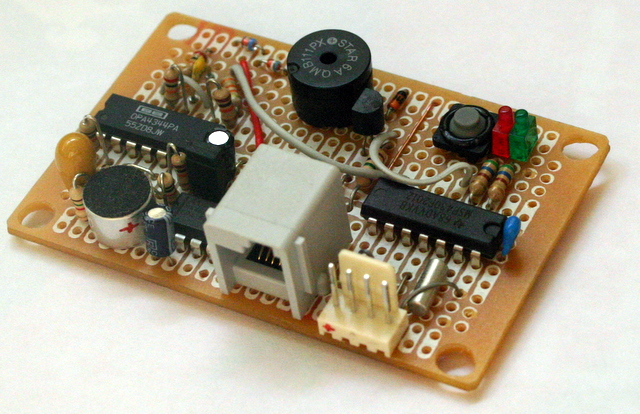The circuit in the picture is a sound sensor that measures both the overall strength of sound and the amount of audio energy in a specific frequency of up to 4kHz. This allows it to detect whether a tone of a particular frequency is played: it compares the audio energy in that frequency to the total amount of energy, and if the ratio is high enough, say above 1/8, it concludes that somebody is playing the tone.

The device can also produce a fairly strong tone at a 2048Hz using a small electromagnetic sounder (the kind that's used in alarm clocks, ovens, etc.). That way, several robots each equiped with such devices can communicate audibly: when one produces a tone, the others can detect it. This should allow them to communicate via Morse code, for example (and would allow people to hear this communication).

Almost all the processing in the device is done digitally. This includes both the detection of the tone and the generation of an frequency-accurate tone. The analog sections are very simple.

The device communicates with a master (a NXT) via I2C. The master can tell it to start and stop producing sound, it can tell what frequency to detect. The device can report to the master the total amount of sound energy in the last period of sampling (right now about 1/40 of a second) and the amount of energy in the frequency it is tuned to.

Warning: connecting your NXT to any home-made gizmo (like the one described here) can damage it. Beware.

## Overall Design

The overall structure of the system is shown in the figure below. The sound is picked up by a microphone and amplified (the right-pointing triangle). It is then passed through a low-pass filter that serves as a simple anti-aliasing filter. This signal is sampled by the analog-to-digital converter (ADC) of the microcontroller, an MSP430F2012 from Texas Instruments. The signal is sampled at 32768 samples per second. These sampled are passed through a digital low-pass filter that produces 8192 samples per second at its ouput.

These 8ks/s samples are processed by an algorithm called the Goertzel algorithm (the box marked G in the figure). This algorithm computes a single Fourier coefficient. This coefficient tells us how much energy the input signal had at a particular frequency. The main useful feature of this algorithm is that it does not need to store the samples; it processes them one at a time. This allowed me to implement the algorithm on a processor with only 128 bytes of memory. A full FFT would have required the storage of all the samples, which is impossible on this processor (and might have been too slow to process the samples in real time). Only two numbers which can be stored in RAM, determine the center frequency of the Goertzel algorithm, which allows the  I2C. master to send the numbers for arbitrary frequencies.

The samples are also integrated in order to measure the total sound energy in the signal. This allows us to compare the sound energy at a particular frequency to the total amount of energy in the signal.

To generate a tone, we use a pulse-width modulation (PWM) output of the processor. The PWM peripheral is programmed to generate a 2048Hz square wave with a 50% duty cycle (high for 50% of the cycle). This signal is fed to a switching transistor (shown as an amplifying triangle in the figure) that turns an electro-magnetic on and off.

The F2012 communicates with the NXT through an I2C interface. To program the chip (and to debug the program running on it), the circuit is connected to an ez430 USB programmer though a home-made extension cord.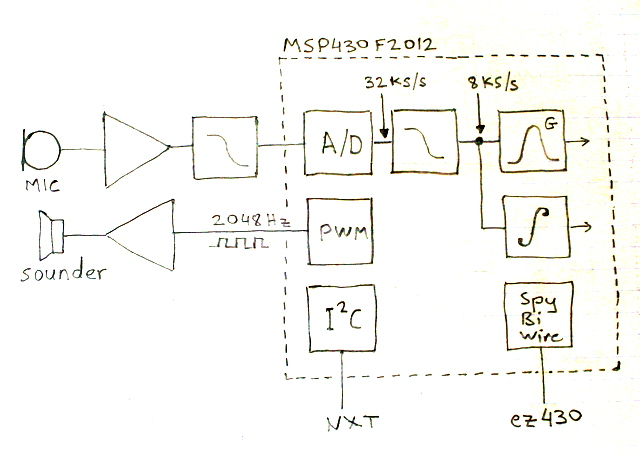The system also contains a few more components not shown in the block diagram. A Maxim MAX604 produces 3.3V regulated power from the NXT's 4.3V supply line. A 32kHz crystal is used by the F2012 for accurate timing of the PWM signal and of the ADC sampling. Two LED's provide visual feedback of tone detection and program failure, and a push switch also helped in the development of the program. The LED's and the switch are connected to the F2012; the LED's through 560Ω resistors and the switch directly.

## The Goertzel Algorithm

The key element in the design is the Goertzel algorithm, which computes a single Fourier coefficient.

Periodic signals can be described as a time series of amplitudes, but also as a linear combination of sines and cosines. for example, the figure below shows a periodic signal. We can represent it as a time series x(t), where t is time, or as a sum of two sines, x(t)=sin(3t)+sin(10t).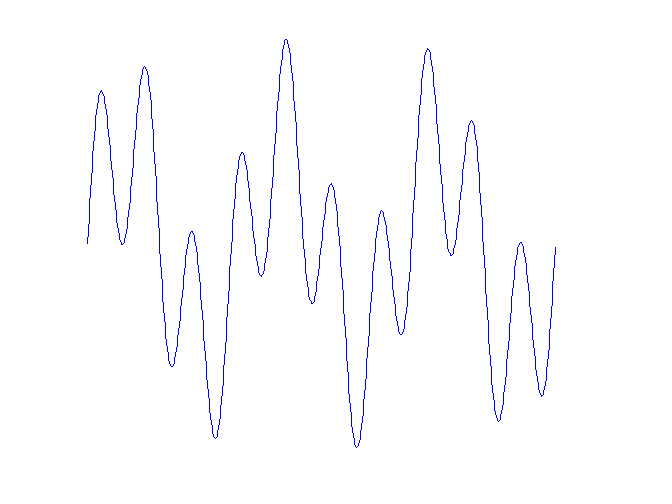A Fourier transform is a mathematical operation that takes a signal (continuous or sampled) as a time series and represents it as a linear combination of sines and cosines at particular frequencies. The fast Fourier transform (FFT) is a family of particularly efficeint algorithms that computes all the Fourier coefficients of a sampled signal. The FFT is not appropriate for our purposes. First, although it is efficient, it requires more work (arithmetic operations) than computing a single Fourier coefficient. Second, it requires us to store all the samples; the F2012 does not have enough RAM to store tham. Third, it works particularly well on sample sizes that are powers of two and it produces Fourier coefficients for equidistant frequencies (0, 1, 2, 3, and so on). This reduces its flexibility: if we want the Fourier coefficient of 1532Hz from 197 samples of a signal, the FFT is not a good computational tool.

The Goertzel algorithm is a clever way to compute a single Fourier coefficient. It can compute the coefficient of any frequency from a sample size of any size. It uses only two multiplication per sample, and it only needs one sample at a time. Because it only uses one sample at a time, in sequence, it can process the samples as they come. Once a sample has been processed, we don't need to store it any more. The algorithm uses only a few variables. Some of them carry information from one sample to the next, and two of them are fixed numbers (a sine and a cosine) that determine the frequency of the coefficient that is computed.

## Windows

To use the Goertzel algorithm, or most other signal processing algorithms, we chop the infinite stream of samples into fixed sized windows and run the algorithm on each window. In Fourier transforms, the size of the window determines the frequency range of the response. In the figure below, we show the magnitude of the Fourier coefficient of 2048Hz for pure-sine signals of frequencies ranging from 0 to 4kHz. The window size is 50 samples.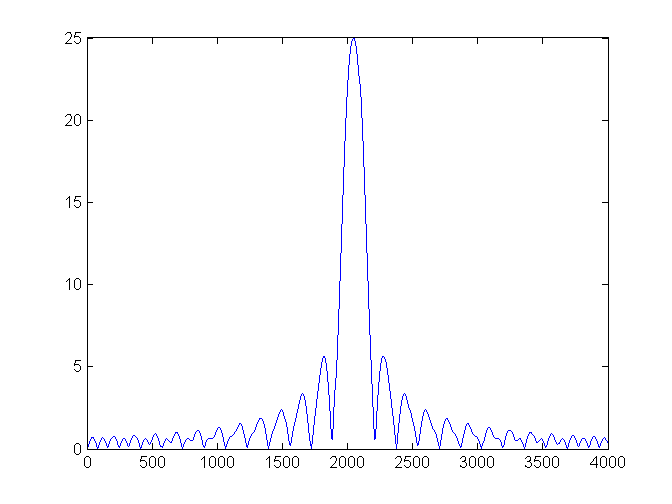If we process the same signals using a window of 200 samples, the response becomes much more localized.This is better if we want to detect a very specific tone. The main down side of using more samples is that it will take us longer to detect the tone.

Both of these response curves show that the Fourier coefficient responds not only to the frequency it is tuned for, but to many other frequencies as well, even very far from the tuned frequency. The response weakens as we get further away from the tuned frequency, but it does not decay quickly. The reason for this is that most of the signals that we tested are not periodic when chopped into a window of 200 samples. To get a fast decay, we need to coerce the signal that we have in the window into a periodic one. This is done by scaling down all the samples. The samples at the endpoints of the window are scaled almost to zero. There are several formulas for such scalings; I used a formula called a Hamming window. Here are the scaling values for a window of 50 samples.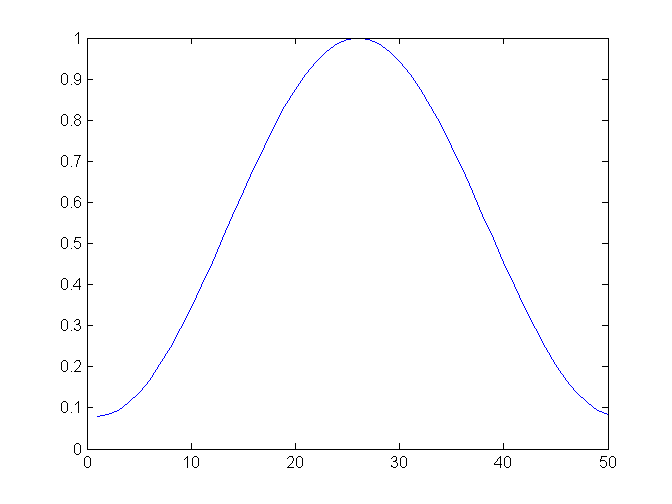If we apply the Hamming scaling window to our signals and then compute the Fourier coefficient of 2048Hz, we can the following response curve.You can see that the decay to zero away from the center frequency is much stronger, but on the other hand the coefficient responds to more frequencies near the center. This is the price for the decay to zero further away.

Using the Hamming window requires storing its coefficients. They are stored in a constant array, so they reside in flash, not in RAM. Flash is much larger than RAM on the F2012 (and on most other microcontrollers), so we can store the coefficients for a large window. The window coefficients depend only on the window size, not on the frequency that we want to detect. Therefore, the same window coefficients are used even if we change the center frequency dynamically.

The computation cost of using a Hamming window is one multiplication per sample.

## Norms

The amount of energy in the input signal is the square root of the sum of squares of the samples. This is also called the two-norm of the signal. But computing it requires one multiplication per sample, and if we accumulate the squares, the numbers involved are large. Therefore, I approximated the energy by the sum of squares of the samples. This is less accurate, but should usually be good enough.

Similarly, the energy represented by a Fourier coefficient is the absolute value of the coefficient, which is a complex number. I again approximated this by the sum of squares. This introduces some artifacts in the response curve, but it is good enough for us, and it is faster. Here is the response curve.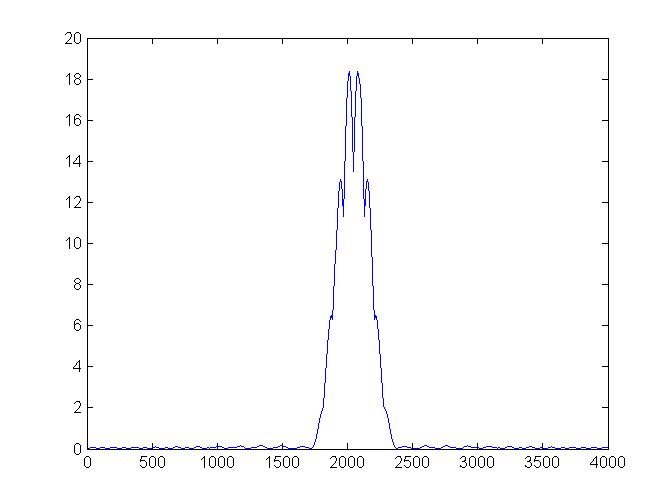## Fixed Point and DSP without a Multiplier

The response curves that we have seen up to now computed the Fourier coefficient using floating-point arithmetic on a PC. Microcontrollers do not support floating-point in hardware, so if you implement the algorithm using floating point (`float` or `double` in C), it will either not compile, or it will compile but run very slowly. So we need to use integer arithmetic. Fourier analysis requires fractional numbers, so we will represent them using integers by imagining that the binary point is in the middle of the number, not to the right of the rightmost digit. This is called fixed point arithmetic.

The registers of the MSP430 processors are 16-bit wide, so arithmetic on 16-bit integers runs much faster than on 32-bit integers. Nonetheless, it is hard to implement algorithms with small word sizes. If you place the binary point too much to the right, you loose accuracy. If you place it too much to the left, you get overflows, which are really catastrophic. When an overflow occurs in a fixed-point algorithm, the results are usually completely wrong. In Fourier algorithms, one of the things that determine the largest numbers that show up in the algorithm is the length of the window. As the window grows larger, the Fourier coefficients (and intermediate results) get larger. The other thing that determines the range of numbers is the accuracy of the ADC; the more bits it provides in each sample, the larger the integers in the implementation of the signal-processing algorithm.

It is probably possible to implement the Goertzel algorithm on the F2012 on useful window lengths in 16 bits, but it is hard. I did a few experiments and decided that it was really on the border of what you can do with 16 bits. On small window sizes I got good results, but on longer the algorithm overflowed and produced junk. Therefore, I decided to use 32-bit integers. This really slowed down the algorithm, but it can stil handle more than 8000 samples per second. With 32-bit arithmetic, you can safely use large windows (I use 200).

The arithmetic unit on the MSP430 processors is pretty weak. It only operates on 16-bit numbers, so operations on 32-bit integers require several instructions (the C compiler generate them automatically). Some of the high-end MSP430's have a hardware multiplier, but the F2012 does not. Therefore, multiplication is performed using a library function that uses repeated addition. This is really slow. I used the simulator that comes with one of the free development environments (IAR Embedded Workbench) to see how long 32-bit multiplications take. I observed running times between 100 and 300 cycles. The running time depends on the number of leading zeros in the first multiplicand, so it helps to try to get the first multiplicand to be positive and smaller. For example, to multiply a sample by a Hamming coefficient, you should place the Hamming coefficient first in the multiplication.

The algorithm performs 3 multiplications per sample and a few other operations (mainly additions and shifts). It can keep up with 8000 or 8192 samples per second, but just barely. I run the processor at 16MHz, which means that the algorithm has a bit less than 2000 cycles to process each sample. I added to the code a conditional that lights a red LED if it fails to keep up with the incoming cycles. If I order multiplications so that the smaller number is the first multiplicand, the processor can keep up. But if I flip the order of multiplications by the Hamming window, it fails to process the samples on time.

## Anti-Aliasing

Digital signal processing (DSP) provides correct results only if the highest frequency component of the signal is at most half the sampling frequency. Higher frequencies in the analog signal look like lower-frequency components that are not really present in the signal. This phenomenon is called aliasing and you need to minimize it in order to get useful results from a digitized signal.

To avoid aliasing, you need to filter high-frequencies from the signal. This filtering is called anti-aliasing. Some of this filtering must be done in an analog circuit, because one you sample the signal, you cannot remove antialiasing that has already occured.

Ideally, we would use a filter that does not attenuate or distort frequencies below half the sampling frequency fs, but that completely cuts out frequencies just above fs/2. Unfortunately, there are no such filters. The attenuation of simple low-pass filters increases with frequency only mildly, so to avoid antialising, you need to start filtering at a frequency much smaller than fs/2. More sophisticated analog filters have a more steep dropoff, but they are harder to design and build. The most common filters, called active filters, require a lot of accurate and stable resistors and capacitors if you want to get a steep drop off. Another kind, called a switched-capacitor filter is much easier to use, but requires a clock that must be synchronized with the clock of the analog-to-digital converer. Therefore, it is somewhat challenging to interface.

I decided to use an anti-aliasing approach that is partially analog and partially digital and easy in both domains. It was suggested in two application notes from Texas Instruments that show how to sample audio on the MSP430.

It works like this. The analog part of the system only includes a simple low-pass filter (I used just a single stage RC filter; you can use more stages and/or some other filtering scheme). The goal of this antialiasing is to pass signals below 4kHz (the pass-band) and to filter signals above 16kHz (the stop-band). We don't care about signals between 4kHz and 16kHz, as long as they are not amplified. This lax specification of the filter is what allows us to use a simple filter.

We sample the signal at 32768 samples per second. This is why we need to filter well anything above 16kHz. There is no point in running the Goertzel algorithm at this rate (even if the processor could do it, which it cannot, at least not in 32-bit fixed point), because we do not care about very high frequencies. What we will do is to sum every sequence of 4 samples and treat the sum as a single sample. We have 8192 such sums per second, which is the rate at which we want to run the Goertzel algorithm. The summation is a form of low-pass filtering. You can think about it as computing averages, but without dividing by 4. We avoid the division by 4 to increase the effective resolution of the ADC.

The reason that the digital low-pass filter can handle 32ks/s whereas the Goertzel algorithm cannot is that the low-pass filter is much simpler. It only involves one 16-bit addition, so it takes only a few cycles. My program performs this summation in the interrupt routine that the ADC invokes. Every 4 samples the interrupt routine puts the sum in a buffer (and lights the red LED if the buffer is not empty) and wakes up the processor from sleep, which causes it to process the sample.

## The Analog Amplifier and Low-Pass Filter

The analog input section is built around a quad opamp, OPA4344. This is a low-voltage rail-to-rail opamp. The exact specifications are not critical in this circuit, but the ability to swing the output all the way at low supply voltages is useful, because it allows the ADC to use its full range.

I used all the 4 opamps in the package, but the circuit is really almost as simple as it gets. I used three opamps in identical inverting-amplifier configurations, each with a gain of 10. The fourth was used to generate the mid-supply bias voltage for the other three. The RC low-pass filter sits between the output of the last amplifier and the ADC input pin of the MSP430. The only other components are an electret microphone connected by a 1.5k resistor to the 3.3V supply and by a blocking capacitor to the input of the first amplifier.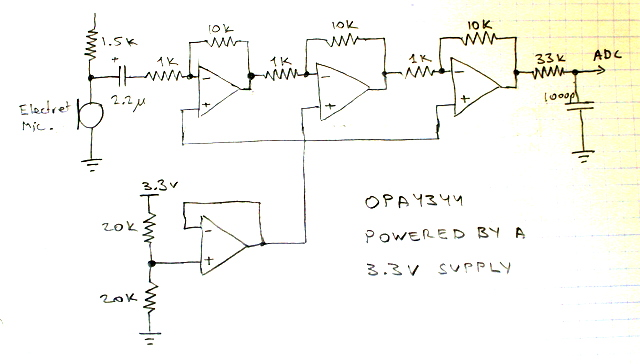It should be possible to feed the ADC using one or two opamps, but I had a quad package so I used all the opamps in it. I also wanted to keep the circuit simple enough that I would understand it completely. I am sure that it is possible to build much better analog sections from the 4 opamps in the OPA4344.

## Producing Sound

The device can also produce sound. My objectives were to produce a strong sound with most of the energy at a fixed precise frequency that other similar devices would be able to detect. Although there are pretty small speakers, a speaker is not a good choice for such a device. A speaker is designed for fidelity. Therefore, to get most of the energy at a single frequency you need to feed the speaker with a sine wave. A microcontroller is not good at producing sine waves; it is good at producing square waves.

There are several ways to produce sine waves. One is with a separate oscillator. It would require tuning to get the correct frequency. Another option is to let the processor produce a square wave and to pass the signal through a low-pass filter (a sqaure wave is a sum of sines in the fundamental frequecy and its harmonics; we need to filter the harmonics). In either case the sine would require amplification to produce a strong sound from a spkeaker.

A better option is to use a device called a sounder. There are two kinds: piezo sounders and electro-magnetic ones. I used an electro-magnetic sounder. These are basically tiny speakers. They have a response curve with high peaks, so they do not reproduce sound accurately. Instead, they tend to resonate mechanically when excited. You can excite them with a square wave and still get a very clean sine-wave audio output.

The sounder I used is called QMB111PX from Star Micronics. It is designed to resonate at 2048Hz, but tends to resonate at 4096Hz. When I drive it with a 4096Hz square wave, it produces a very clean sine output. But I do not want a 4096Hz output because it is at exactly half the sampling frequency. I want a 2048Hz sound. When I drive it with a 2048Hz it produces a combination of 2048Hz and 4096Hz. How much of each depends mostly on how the sounder is mounted. The volume of sound also depends on the mounting.

The MSP430 cannot drive the sounder directly. I used a MOSFET transistor (BS170) to switch the sounder. You also need a reverse-biased diode across the sounder, to protect the transistor from the inductive load of the sounder. The sounder that I used is designed for 15-2V, so I used 3 diodes in series to drop about 1.8 of the 3.3v supply voltage before it hits the sounder.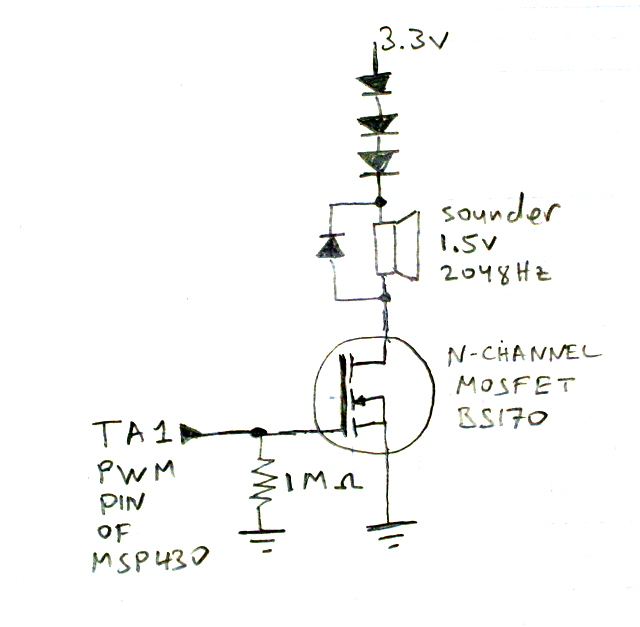It is possible to replace the MOSFET transistor by an NPN transistor. It would draw some base current from the MSP430, but it works. (You need a current-limiting resistor between the pin of the MSP430 and the base of the transistor.)

I am hoping to find better sounders. There are 3 ways to do better than the QMB111PX: (1) a sounder that does not produce a strong harmonic at 4kHz, (2) a sounder that runs directly on 3.3V without the need to drop down the voltage to 2V, and (3) a sounder that uses less power, perhaps a piezo one. I'm investigating.

## Construction

I constructed the circuit on a Radio Shack prototyping board. The sides of the board are multiple of 8mm, but the mounting holes are too small for Technic axles/pins, and their centers are a bit too far apart. I enlarged the mounting holes (very carefully); they still don't fit Technic axles, but at least they fit Technic pins, and at the right center-to-center distances.The chip on the right is the MSP430F2012, the one on the left is the quad opamp, and the one near the NXT socket is the MAX604 voltage regulator. The small silver cylinder is the microphone and the larger black one is the sounder. I fit a NXT socket on the board. I had to bend the pins a bit, but it worked. I drilled holes (with a manual drill) for the mounting studs of the socket, so it is really secure. You can see them at the top center of the next figure.The processor that I used is in a DIP package, not on a daughter board of the ez430. To program and debug it, I constructed an extension cord for the ez430. I removed all the components from one daughter board (including the processor) and soldered instead a 4-wire flat cable. It connects to 3 of the ez430's terminals directly, but to the 3.6V power connector through a jumper. This allows me to connect the ez430 to a circuit that runs on its own power.

I initially left the ez430 and the daughter board with the extension cord exposed, without the plastic cover. At some point, my ez430 died completely. I am not sure why, but perhaps it was damaged by static electricity or by a metal object that caused a short. I bought another and decided to keep it enclosed. I modifed the plastic enslosure to allow the extension cable out and to allow accesss to the jumper pins. So far it works.This contraption allows me to build circuits with an MSP430 and to program and debug them in the circuit. The 4 wires from the ez430 connect directly to the chip. A pull-up resistor on the reset pin ensures that the chip boots once power is applied when the ez430 is not connected (when it is, it prevents the chip from booting).

A MAX604 produces 3.3V from the NXT's 4.3V. To allow the circuit to run with power from the ez430 (or from a pair of AA batteries; it still detects the tone and lights a green LED when it does!), I connected the output pin of the MAX604 to the rest of the circuit through a jumper.

In the pictures below you can see the extension cord that I built for the ez430, an F2012 in a breadboard ready to be connected to the ez430, and the F2012 connected to the ez430.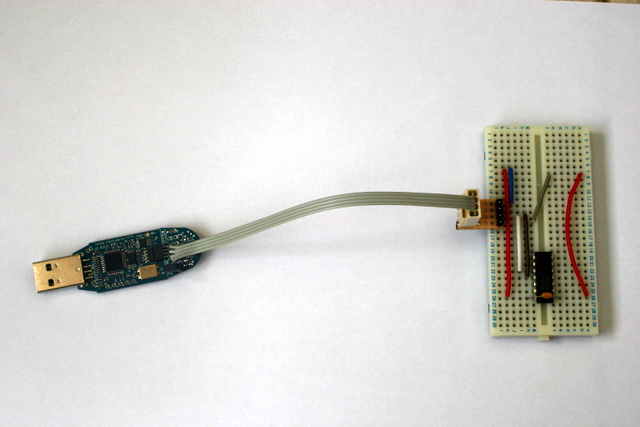## Algorithm Prototyping with a PC

The algorithm that the MSP430 runs is not trivial to get right, especially when fixed-point arithmetic is used. I didn't try to develop the algorithm directly on the MSP430. Istead, I first developed a version in Matlab (a numerical computing environment). The graphs that appeared above were produced by this prototype. I first developed the algorithm in floating point. When it was working, I created a fixed-point version and explored its behavior. I first tested the codes on synthetic sine waves produced in Matlab, and then on recordings of actual sounds from a buzzer (similar to a sounder, but with an internal circuit, so it produces sound when connected to DC).

When the Matlab program was working properly, I converted it to a C program for the MSP430. Every time I optimized the MSP430 program, I made a similar change in the Matlab program to ensure that I still get correct results.

I also used Matlab to analyze the audio from the sounder and from other devices that I tried, to assess whether they produce the right frequency and to assess how clean the sound is. To do that, I wrote a little Matlab program that recorded a bit of audio from the laptop's microphone, then computed the FFT of the result and presented it graphically, then repeating.

## The Tradeoffs associated with the Crystal Oscillator

The F2012 has several oscillators. They are used for clocking both the processor and the peripherals, including the timers. One is a digitally-controlled oscillator (DCO) that can be set to 1, 8, 12, or 16MHz, the second is a 32kHz crystal oscillator, and the third is a very low-frequency oscillator that is irrelevant for this design.

I clocked the timer from the 32kHz (more precisely, 32,768Hz) oscillator, which requires an external crystal. This allows the system to sample at an accurate rate, so its center detection frequency is both stable and accurate, and the tone that it produces is at an accurate frequency.

The main disadvantage of this setup is that the 32kHz does not allow you to sample at a rate higher than 32kHz and does give much flexibility in the tone that it generates. Even sampling at 32kHz is hard; I had to use two different interrupt routines to wake up the ADC (sampling at 8 or 16kHz is easy).

If you connect the timer to the DCO, which I run at 16MHz to do the signal processing fast enough, you have a much more flexibility in choosing the sampling rate and in terms of generating tones. In particular, it should be possible to sample at 64 or even 128ks per second, which would improve anti-aliasing even further. The disadvantage of using the DCO is accuracy; its frequency is only accurate to within 6%, if you take into consideration calibration errors and frequency dependence on temperature and supply voltage. This is a pretty wide range of possible clock frequencies, which would make reliable tone detection difficult.

Perhaps the best design is to use the DCO to clock the timer, but to calibrate the timer using a crystal oscillator. The F2012 has two timers; a general-purpose timer and an interval/watchdog timer. You would use the DCO to clock one and the 32768Hz crystal oscillator to clock the other. This would allow you to use the crystal oscillator to determine the exact rate at which the DCO oscillates. It might work, but it's a pretty complicated approach.

Many other MCU's in the MSP430 family (as well as in other MCU families) can be clocked by a high-frequency (several MHz) crystal oscillator, which would eliminate this tradeoff altogether.

## Software

Here is the software that I wrote for the device:

• The Matlab program that generates the coefficients and which tests the algorithm. This file is not cleaned up.
• The C program that runs on the MSP430F2012 and the file with the coefficients. The program starts by repeatedly performing the Goertzel algorithm and displaying the result using a green LED. It also listens as a slave to the I2C channel. If the master tries to read, it sends 9 bytes: two 32-bit integers representing the overall sound energy and the sound energy near 2048Hz, and a one-byte sequence number of the measurement. The sequence number allows the I2C master to know whether it is reading the same measurement twice. The I2C master can also send the device 3 commands: to start sounding a 2048Hz sound, to stop the sound, and to start monitoring sound again. You can also start and stop the sound from the button on the device itself. The code should compile under both IAR Embedded Workbench and under Code Composer Essentials (but my final tests where under IAR, which seems to produce much more compact code).
• The RobotC program that runs on the NXT as an I2C master. The program repeatedly reads the data from the device and displays it, but if you press the center (orange) button it tells the device to sound a tone instead. When you release the button, the sound stops and sound monitoring resumes.
• An NXC program that does the same thing. It does not run correctly under the standard firmware (version 1.04), because it has bugs in multibyte I2C transactions. It runs without a problem with John Hansen's improved firmware.

## An Alternative Analog Design

When I first thought of building an audio two-way signaling device for the NXT, I thought of using mostly analog devices, not digital signal processing.

The LM567 is a single-purpose chip that detects whether a single frequency is presentin the signal that you feed it. It runs on 5V, and there is a 3.3V version called the LMC567. You program the frequency that you want to detect using resistors and capacitors. It should be fairly easy to build a device similar to mine around this chip. You would still need a microphone amplifier, but that's pretty much it. The LM567 produces a digital output present/not-present signal that you can communicate to the NXT using an I2C interface chip, or simply through the analog sense line. To produce the tone, you could use any oscillator circuit. This analog device would not be as accurate as my device (my devices accurate depends on that of a crystal oscillator, which is good), and it would not be easy to change the tuned frequency. You could change it with a trimmer, of course, but it would be hard to change it from the program. One way to detect multiple frequencies with the LM567 is to use several of them, each tuned to a different frequency. Another way is to use an I2C potentiometer to adjust the center frequency.

The cost of this solution is a little lower than that of mine, but not by much: Digikey charges \$1.80 for the LM567 (\$1.89 for the LMC567) and \$2.70 for the MSP430F2012. So the versatile MCU is more expensive than the special-purpose analog chip, but not by much.

The main advantage of the analog solution is that it is probably a lot easier to develop and to debug, because there is no software involved.

## More on Parts

If you build something like this, you may be able to use parts salvaged from broken or obsolete circuits. Electromagnetic sounders are fairly common. In my home we had 6 or 7 dead analog alarm clocks, and all of them had sounders similar to the one I used. PC motherboards also use these devies. If you use a salvaged sounder, check whether it indeed produces the tone that you need. 32768Hz crystals are also common in battery-operated alarm clocks and in motherboards. The crystal the I used is from a dead motherboard.

The opamp that I used (OPA4344) is a pretty expensive one, at more than \$4 at Digikey, but you can build a similar circuit from cheap opamps like the LM324.

Finally, I used a 3.3V regulator in the circuit, but neither the MSP430 nor the opamp need a regulated supply. The MSP430 needs a supply voltage of at most 3.6V, so you can't power it directly from the 4.3V supply of the NXT. But you can use a Zenner diode or a few common silicon ones in series to get the voltage to the correct range. If you use a higher supply voltage for the opamp than for the MSP430, make sure that the analog input to the MSP430's ADC does not exceed the supply voltage of the MSP430.